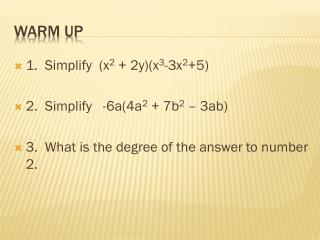DownloadDownload PresentationWarm up

# Warm up

Download Presentation## Warm up

- - - - - - - - - - - - - - - - - - - - - - - - - - - E N D - - - - - - - - - - - - - - - - - - - - - - - - - - -
##### Presentation Transcript

1. 1. Simplify (x2 + 2y)(x3-3x2+5) 2. Simplify -6a(4a2 + 7b2 – 3ab) 3. What is the degree of the answer to number 2. Warm up

2. Objective: To review all previously learned methods of factoring Factoring

3. Factoring a common term (monomial) • When a polynomial has a factor (either a constant or a variable) that is common to all terms it should be the first thing factored out of the polynomial. • (x3 + x) factors to x(x2 + 1) Factoring

4. 4x2 –x 3x4 – 9x2 3m(2x-3y)-n(2x-3y) Factoring

5. Factoring by Grouping – sometimes once you group the terms of the polynomial you will find that you can then factor out common terms. • 3xy+2y + 3xz +2z try grouping the terms containing y together and the terms containing z together • (3xy + 2y) + (3xz + 2z) factor out common terms y(3x +2) + z(3x + 2) factor out common binomials (3x+2)(y +z) Factoring

6. Try: • 2m3n + m2 + 2mn2 +n • 2a2– 4ab2– ab + 2b3 Factoring

7. Factoring Second Degree Polynomials • Polynomials in the form x2 + bx + c factor to 2 binomials (x + __)(x + __) • The last numbers in the binomial are found by finding the factors of c that add up to b. • Ex: x2 + 7x +12 1 12 = 13 2 6 = 8 3 4 = 7 Factoring

8. x2 – 7x + 10 x2 – 3x - 4 Factoring

9. Factoring Second Degree Polynomials • Polynomials in the form ax2 + bx + c factor to 2 binomials (_x + __)(_x + __) • You must have factors of a before x and factors of c at the end of each binomial. • Ex: 3x2 + 9x + 6 (3x + __)(x + __) look for factors of 6 that will make the outer and inner multiply to 9. (3x + 3)(x + 2) factoring

10. Another approach to that type of polynomial: • 3x2 + 11x + 6 Put the 3x at the beginning of (3x +__)(3x +__) each binomial Now multiply the 6 by 3 - find factors of 18 that add up to 11 (3x + 9)(3x + 2) Now the first binomial can be factored again, making it (x + 3) so that is what the final factor should look like: (x + 3)(3x + 2) Factoring

11. Try: • 3x2 – 16x + 21 • 2x2 + 3x – 9 Factoring

12. Difference of Two Squares a2 – b2 • Factors to (a + b)(a-b) • Ex: x2 – 25 (x + 5)(x – 5) Factoring

13. 9s2 – 49t2 x4 - 1 Factoring

14. Sum & Difference of Two Cubes • a3 + b3 = (a + b)(a2 – ab + b2) • a3 – b3 = (a – b)(a2 + ab + b2) • Ex: x3 + 8 (x + 2)(x2 – 2x + 4) x3 – 27 (x – 3)(x2 + 3x + 9) Factoring

15. x3 – 1 64m3 + 125 Factoring

16. All these methods could be combined: • 2x3 – 8x factor out a common monomial • 2x(x2 – 4) difference of 2 squares • 2x(x-2)(x+2) once you are sure that each factor is prime – you are done Factoring

17. x3 + 5x2 – 6x 2x3 – 2x2y – 4xy2 -3x(x+1) + (x + 1)( 2x2 + 1) Factoring